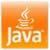# Java program to calculate area of Triangle - Homework, programming exercise example

Calculate the area of Triangle in Java
Write a Java program to calculate Area of Triangle, accept input from User, and display output in Console is one of the frequently asked homework questions. This is not a tough programming exercise but a good exercise for beginners and anyone who has started working in Java. For calculating the area of a triangle using formula 1/2(base*height), you need to use arithmetic operators. By doing this kind of exercise you will understand how arithmetic operators work in Java, subtle details which affect calculation like precedence and associativity of the operator, etc.

Fortunately this question is not very popular on Java interview like other exercises we have seen e.g. Java program to print Fibonacci series and Java program to check for palindrome. Nevertheless, it's a good Java homework.

Also, basic knowledge of essential data structure and algorithms is also very important and that's why I suggest all Java programmers join a comprehensive Data Structure and Algorithms course like Data Structures and Algorithms: Deep Dive Using Java on Udemy to improve your knowledge and algorithms skills.

## How to calculate the area of Triangle in JavaIn this section, we will see a complete code example of How to calculate Area of Triangle in Java. We are going to use classic formula 1/2(base*height), where base and height will be accepted as user input using java.util.Scanner class. The arithmetic operator used in this exercise is multiplication and division i.e * and /.

/**
*
* Java program to calculate area of Triangle .
* Formula for calculating area of Triangle is 1/2(base*height)
*
* @author Javin Paul
*/

public class AreaOfTriangle {

public static void main(String args[]) {

Scanner scanner =
new Scanner(System.in);

System.
out.println("Please enter base width of Triangle ");

float base = scanner.nextFloat();

System.
out.println("Please enter height of Triangle ");

float height = scanner.nextFloat();

//calculating area of Triangle in Java

float area = area(base, height);

System.
out.println("Area of Triangle calculated by Java program is : " + area);

}

public static float area(float base, float height){

return (base* height)/2;

}
}

Output
Please enter base width of Triangle
20
30
Area of Traingle calculated by Java program is :
300.0

That's the whole Java program to calculate area of triangle and Now let me introduce with one of the most common mistake Java programmer make while writing this code. If you look closely to area() method you will find that we have wrapped code into small bracket i.e. (base* height)/2 . We have done that to increase precedence, anything which is inside bracket will be executed first. Just change the code, like you write in notebook e.g. 1/2*base*height and suddenly your calculate area for triangle become zero, as shown below :

public static float area(float base, float height){
return 1/2*base*height;
}

Please enter base width of Triangle
10
40
Area of Traingle calculated by Java program is : 0.0

For a beginner in Java,  this is not an easy bug to find or spot on. In order to find the bug you must know how Java evaluates an arithmetic expression. Here two arithmetic operator * and / are used which are right associative i.e. evaluated from right to left and of same precedence.

first expression which is evaluated by Java is 1/2 which is division of two integer and result of this would be an integer i.e. zero and not 0.5, which is making whole area zero. This is happening because  of both operands of division operator(/) is integer, if any of them is float or double then result would be a floating point number. by combining (base*height) in bracket we ensure that it executed first and produce a floating point number which can be divided by two.

This kind of simple Java mistakes can only be avoided by learning and writing such kind of program. That's why programs like How to calculate square root in Java should be in list of Java learner.

That's all on How to calculate area of Triangle in Java. We have seen complete code and also analyzed possible but and How arithmetic expression is evaluate in Java especially multiplication and division operator.

Further Learning
The Coding Interview Bootcamp: Algorithms + Data Structures
Data Structures and Algorithms: Deep Dive Using Java
Algorithms and Data Structures - Part 1 and 2

Other Java programming and homework exercise

Unknown said...

Awesome code.... good thinking..... Now I realise why area always came 0.0..... but sir,
I then tried (double)(1/2)*base*height; but still it is coming 0.0.... can you tell me why is it coming like that?

Anonymous said...

how do you calculate area of triangle if only co-ordinates of vertices are given and no base or height is provided?

javin paul said...

You can use following formula to calculate area of triangle with co-ordinates of all three vertices given

area = (x1(y2 - y3) + x2(y3 - y1) + x3(y1 - y2))/2

Unknown said...

import java.util.Scanner;

/**
* Calculates area of triangle in Java given its base and vertical height.
* @author jj
*/
public class AreaOfTriangle1 {

public static void main(String[] args) {

Scanner sn = new Scanner(System.in);
System.out.println("Enter base of triangle:");
double base = sn.nextDouble();

System.out.println("Enter vertical height of triangle:");
double verticalHeight = sn.nextDouble();

AreaOfTriangle1 at = new AreaOfTriangle1();
double area = at.calculateArea(base,verticalHeight);

System.out.println("Area = "+area);

}

/**
* Calculates area of a triangle using base and vertical height
* @param base
* @param verticalHeight
* @return
*/
private double calculateArea(double base, double verticalHeight) {
return base*verticalHeight/2;
}
}

Anonymous said...

When (1/2) is been operated,it's resulting 0.5 and ....the whole part of it ie 0 is been considered by the compiler ,that's why it's returning 0 and 0.0 is because of 'double'